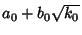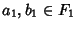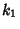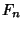## Constructible Number

A number which can be represented by a Finite number of Additions, Subtractions, Multiplications, Divisions, and Finite Square Root extractions of integers. Such numbers correspond to Line Segments which can be constructed using only Straightedge and Compass.

All Rational Numbers are constructible, and all constructible numbers are Algebraic Numbers (Courant and Robbins 1996, p. 133). If a Cubic Equation with rational coefficients has no rational root, then none of its roots is constructible (Courant and Robbins, p. 136).

In particular, letbe the Field of Rational Numbers. Now construct an extension fieldof constructible numbers by the adjunction of, whereis in, butis not, consisting of all numbers of the form, where. Next, construct an extension fieldofby the adjunction of, defined as the numbers, where, andis a number infor whichdoes not lie in. Continue the processtimes. Then constructible numbers are precisely those which can be reached by such a sequence of extension fields, whereis a measure of the complexity'' of the construction (Courant and Robbins 1996).

See also Algebraic Number, Compass, Constructible Polygon, Euclidean Number, Rational Number, Straightedge

References

Courant, R. and Robbins, H. Constructible Numbers and Number Fields.'' §3.2 in What is Mathematics?: An Elementary Approach to Ideas and Methods, 2nd ed. Oxford, England: Oxford University Press, pp. 127-134, 1996.